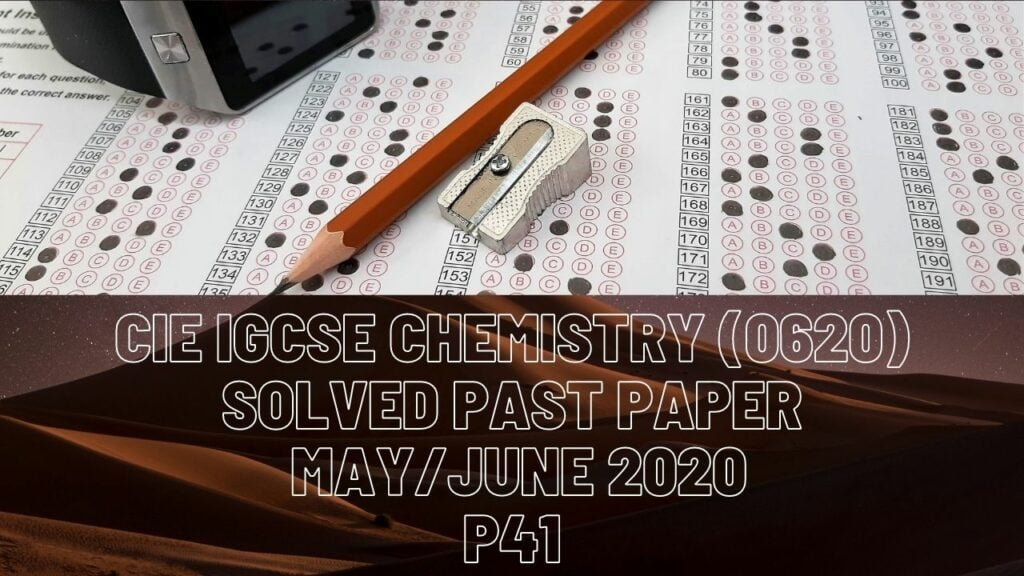IGCSE Chemistry### CIE IGCSE Chemistry Solved Past Paper May/June 2020 P41

1 ai) Protons and neutrons

ii) Nucleon number

iii) 34

iv) 2 8 8

v) Al2O3

bi) Isotopes

ii) Carbon-12

iii) One mole

c) = sum of products of isotopic mass and their respective ratios

= ((69*3)+(71*2))/5

= 349/5

= 69.8

Rounded to 70, the Ar of gallium

di) Phosphorus

ii) It gains 3 electrons

2a) Metallic bonding – enforced by force of attraction between mobile electrons from sea of electrons and positive ions(metal atoms after losing outer shell electrons)

bi) Mg 2+ and O 2- ions with complete outer shells having 8 electrons. Magnesium ion– 2 shells and oxygen ion– 2 shells

ii) 2Mg + O2 2MgO

ci) 2Mg(NO3)2——>2MgO + 4NO2 +O2

ii) Thermal decomposition (reactant broken down to multiple products by heat intake)

iii) Magnesium hydroxide and magnesium carbonate are the compounds

3a) Zinc blend

bi) The reaction is reversible and rate of forward reaction is equal to rate of backward reaction

iii) It increases the rate of the reaction

iv) Molecules gain kinetic energy, they move faster and so their rate of collision increases, more reactant molecules have sufficient energy to react(activation energy = energy required to initiate a chemical reaction).

v) It indicates that forward reaction is exothermic

vi) % composition = (Ar of S/Mr of compound)*100

= (32/(32+3*16))*100

= 40%

c) Anhydrous copper(ll) sulfate. Carbon (this is an exothermic reaction, dehydration of sucrose has the products water and carbon, the heat of reaction vaporises water and leaves carbon as the only product).

di) It has at least one C=C double bond

ii) C3H7OH——>C3H6 + H2O

(Propanol has Mr 60, according to question, one of the 2 products has mass of 42, which means remaining mass is 18 and since this is a dehydration reaction, smaller molecule is water this leaves 3 carbon atoms and 6 hydrogen atoms, which make up propene).

(iii) Propene

4a) Ammonia is tested by damp litmus paper, which turns blue for positive results

bi) Ammonia acting as a proton acceptor is shown here(chemical property of a base)

ii) 9

iii) Blue precipitate forms  in access ammonia, precipitate dissolves giving a deep blue solution.

ci) Neutralisation (acid and alkali)

ii) 2NaOH + H2SO4—–> Na2SO4 + 2H2O

di) Methyl orange

ii) Moles = concentration*volume(in dm cube)

= 0.04 * (25/1000)

= 0.001 mol

2 moles of NaOH give 1 mole of H2SO4 (ratio from equation)  i.e half the number of moles of acid for every single mole of NaOH. Therefore moles = 0.001/2 = 0.0005 mol of H2SO4

Concentration = moles/volume (in dm cube)

= 0.005/(20/1000)

= 0.25 mol/dm3

iii) Convert 0.004 mol to mass here

Mass = moles*molar mass

= 0.004*(23+16+1)

= 1.6g/dm3

5a) Glucose fermentation

Ethene hydration

b) (Warm) acidified potassium manganate(VII)

ci) Condenser

ii) Water goes in from the lower inlet

di) Methanal with the chemical formula C4H8O

ii) CnH2nO

ei) Functional group

fi) Structural isomers